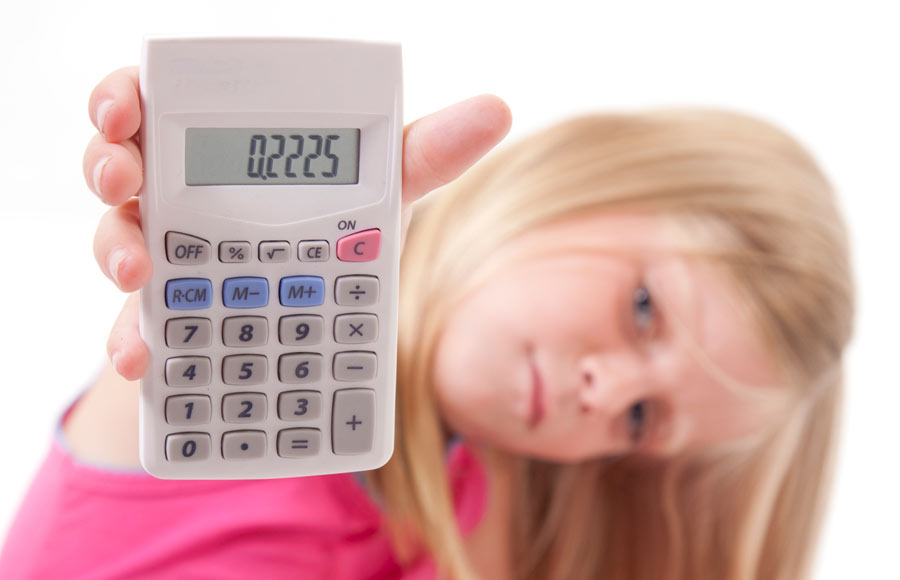# How To Convert a Decimal To a Fraction

| Last update: 12 April 2017

Some decimals are so familiar to us that we can instantly see them as fractions: if your sister is 14.5 years old, you know that she's 14 1/2; if you buy a bag of potatoes weighing 0.75kg, you know that it's 3/4 of a kilo; if you give your sister a 3/4 kilo bag of potatoes for her 18th birthday, you know that your chances of a polite and enthusiastic response are around 0.1, or 1/10.

But what of the other decimals? The cool cats who haven't broken into the mainstream? How can you possibly figure out what 0.65, 0.35 or 0.721 is as a fraction, for example? Well, just put down your potatoes, Janet, because we are going to make it easy for you...## Turning decimals into fractions

The most important thing you need to keep in mind when you want to convert a decimal to a fraction is that a decimal expresses whether something is a 'tenth', a 'hundredth', a 'thousandth' etc., based on its position after the decimal point. If you're looking at a decimal which only has one number after the point, then you are working in tens. If your decimal has two digits after the point, then you will be working in hundreds. If your decimal has three digits after the point, then you are working in thousands, and so on.In this example, there are four digits after the decimal point and so we would be working in ten-thousandths.

## Step 1

Look at your decimal to establish whether you're working in tens, hundreds, thousands or more. This will become your multiplier later on, in step 3.

e.g.:

0.35 is 35 hundredths

## Step 2

Write out your decimal as the numerator of a fraction (i.e. above the fraction line). The denominator below the line is always 1, because a decimal is always part of 1.

e.g.:

0.35/1

## Step 3

Think back to your multiplier from step 1. Multiply your numerator by 10 / 100 / 1000 or whatever your multiplier is, and then do the same calculation for the denominator.

e.g.:

35/100

## Step 4

Now you just need to tidy up your fraction by simplifying it. For this, you need to work out the 'greatest common factor', i.e. the largest number which can be multiplied to make both the numerator and the denominator. This makes your fraction smaller and easier to work with, so always ask your fraction, "does your bum look big in this?" and try slimming it down without changing the proportions.

e.g.:

Both 35 and 100 are multiples of 5, so we can divide both numbers by 5 to keep the proportionate relationship the same.

e.g.:

7/20

So 0.35 = 7/20

Not as painful as having 0.75kg of potatoes thrown in your face, is it?

Of course, if you want to check your answer, we have a handy decimal to fraction calculator that you can make free use of. In addition, we have a great article on how to add, subtract, multiply and divide fractions.

And, hey, if you manage to find a number which simply can't become a fraction, then don't be too hard on yourself. It's not you: it's them. These are called "irrational numbers", and with good reason. One example of an irrational number is pi (3.14159265...) There's just no reasoning with them.